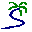# Model entry

Age-class model of population dynamics, one compartment per age class - Model catalogue - Simulistics.com

 Search Simulistics Model catalogue Listed by keyword Listed by ID Listed by title Listed by date added

# Age-class model of population dynamics, one compartment per age class

Model : ageclass4
Simile version : 3.1+
Keywords : Age-class modelling ; Population dynamics ;

## Description

This models a population of animals in terms of four age-classes. For each age-class, we use a compartment (state variable) to represent the number of individuals in that class.

In this model, there are four compartments on the model diagram. They are connected by flow arrows, which represent the aging process: the flow of individuals from one class to the next as they get older. Simile allows the same mathematical model to be represented more concisely, using a single compartment embeddedinside a multiple-instance submodel: see the links to other models below.

## Files

### Model file

Click on the icon to download the model file. (You will need Simile to examine and run the model. A free evaluation version is available from the products page.)

Some browsers may attempt to display the model file, rather than open it in Simile; in this case, use the browser back button to return to this page, and use the context menu (invoked by right-clicking on the link) to save the target file to disk.ageclass4.sml

## Diagram## Equations

```Compartments
pop_size_1: initial value = 2
pop size_2: initial value = 0
pop size_3: initial value = 0
pop size_4: initial value = 0

Flows
ageing_1 = pop_size_1
ageing_2 = 0.2*pop_size_2
ageing_3 = 0.1*pop_size_3
births   = births_2+births_3+births_4
deaths_1 = m1*pop_size_1
deaths_2 = m2*pop_size_2
deaths_3 = m3*pop_size_3
deaths_4 = m4*pop_size_4

Parameters
m1 = 0.05
m2 = 0.01
m3 = 0.01
m4 = 0.05

Intermediate variables
births_2 = r2*pop_size_2
births_3 = r3*pop_size_3
births_4 = r4*pop_size_4
pop size = pop_size_1+pop_size_2+pop_size_3+pop_size_4
r2 = 0.05*(1-pop_size/100)
r3 = 0.2*(1-pop_size/100)
r4 = 0.1*(1-pop_size/100)
```

## Results

||